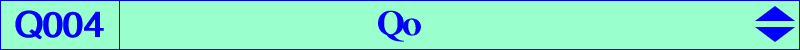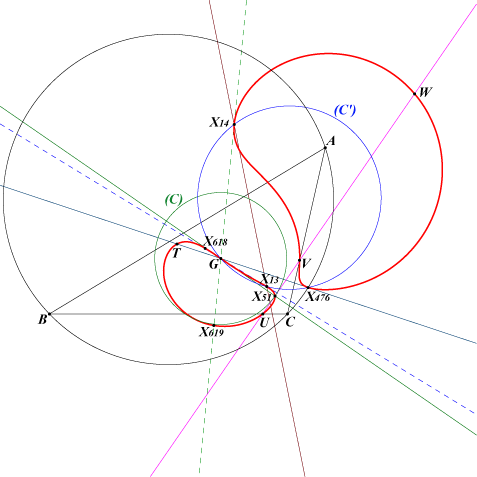too complicated to be written here. Click on the link to download a text file.X(2), X(13), X(14), X(51), X(476), X(618), X(619), X(3258), X(14844), X(14847) T = X(3258) = complement of X(476) traces U, V, W of the trilinear polar of X(1989)Q004 is the locus of the common points of the asymptotes of all pK60+. See Special Isocubics §6.7 and CL006. See the analogous Q153 obtained with nK0s. It is a bicircular quartic with singular focus G, which is at the same time a flex on the curve. The tangent at G is the line GX(51). (C) is the circle centered at G passing through X(51). (C) is tangent at X(51) to the trilinear polar of X(1989). The inversive image of Q004 in (C) is the circular cubic Q005 (denoted by (k) in Special Isocubics §6.7) The tangents at X(13), X(14), X(476) pass through G, these four points lying on the circle (C') which also contains the Parry point X(111). This circle is tangent at G to the Euler line. More generally, the polar line of P in Q004 passes through G if and only if P lies on the central focal cubic K1138. The Psi image of Q004 is a circular cubic with singular focus G passing through X(13), X(14), X(616), X(617), X(2782), X(14651), X(18331), X(18332). Note that the polar conic of G splits into the line at infinity and the Fermat axis.